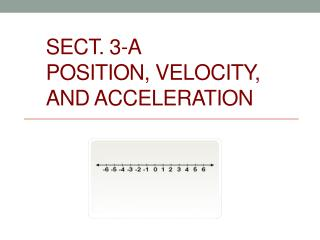# Sect. 3-A Position , Velocity, and Acceleration - PowerPoint PPT PresentationDownload PresentationSect. 3-A Position , Velocity, and Acceleration

Sect. 3-A Position , Velocity, and AccelerationDownload Presentation## Sect. 3-A Position , Velocity, and Acceleration

- - - - - - - - - - - - - - - - - - - - - - - - - - - E N D - - - - - - - - - - - - - - - - - - - - - - - - - - -
##### Presentation Transcript

1. Sect. 3-APosition, Velocity, and Acceleration

2. Position function - gives the location of an object at time t, usually s(t), x(t) or y(t) Velocity - The rate of change( derivative) of position, usually v(t) Acceleration - The rate of change ( derivative) of velocity usually a(t) Average velocity -

3. 1) If find v(t) and a(t).

4. 2) The position of a particle moving along the x - axis at time t is given by . Find the particles velocity and acceleration at t = 5. Velocity Acceleration

5. Speed – The absolute value of velocity otherwise known as the magnitude of velocity

6. Velocity Positive - the particle is moving to the right Negative - the particle is moving to the left Zero - the particle has momentarily stopped or is changing direction ( must have a sign change)

7. 3) Find where the object changes direction if Find where v(t) = 0

8. Acceleration and Velocity • If the sign of acceleration is the same as velocity, the speed of the particle is ______________ (the two are working together) • If the sign of the acceleration is opposite that of velocity, the speed of the particle is _________________ (the two are working against each other)

9. 4) Given the same position function as #3 find the interval during which the particle is slowing down.

10. 5) Given find the interval during which the particle is speeding up.

11. Distance vs. Displacement • Displacement- change in position ( final position minus original position) • Distance- the total distance travelled by an object in the time interval even if duplicated

12. Consider two intervals 6) Find the DISTANCE traveled by the particle whose position is given by on the interval (0,4). Distance NOT displacement!!

13. 7) If • find the DISTANCE traveled by the particle on the interval (2,4). • Find the DISPLACEMENT on the interval (1,5)

14. The graph shows the position function of a radio controlled car a) Was the car going faster at B or at C? b) When was the car stopped? c) At which point was the car’s velocity the greatest? d) At which point was the car’s speed decreasing?

15. Homework PVA Worksheet and worksheet 3-A